## Big Data Hadoop and Spark Developer

Certification Training
26924 Learners
13 Chapters +

# RDDs in Spark Tutorial

Welcome to the eleventh lesson “RDDs in Spark” of Big Data Hadoop Tutorial which is a part of ‘Big Data Hadoop and Spark Developer Certification course’ offered by Simplilearn. This lesson covers the creation of Resilient Distributed Datasets or RDDs and RDD operations.

## Objectives

After completing this lesson, you will be able to:

• Create RDDs from files and collections

• Create RDDs based on whole files

• List the data types supported by RDD

• Apply single-RDD and multi-RDD transformations

In the previous lesson, we have briefly discussed Resilient Distributed Datasets or RDDs as an important feature of Spark. Let us look at the data types supported by RDDs and learn how to create RDDs.

## RDD Data Types and RDD Creation

In the first topic of this lesson, Let us first look at the various data types that RDDs can hold.

RDDs support:

• Primitive Data Types such as integer, character, and Boolean

• Sequence Data Types such as strings, lists, arrays, tuples, and dicts as well as nested data types

• Scala or Java objects which are serializable

• Mixed Data Types

Let us now understand what pair RDDs and double RDDs are.

## Pair RDDs and Double RDDs

Let us look into pair RDD and Double RDD in detail.

What are Pair RDDs?

Spark also provides special RDDs that hold key-value format. These are called Pair RDDs.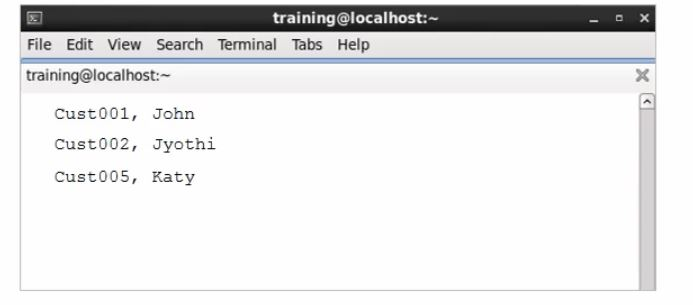Each element of Pair RDDs must be a key-value pair, that is, a two element tuple. The keys and values can be of any type.

Pair RDDs are useful when implementing MapReduce algorithms. Some of the functions that can be performed with Pair RDDs are:

• Map

• FlatMap.

Pair RDDs also support many additional functions for common data processing needs such as:

• Sorting

• Joining

• Grouping

• Counting.

Pair RDDs are discussed further later in the lesson.

What are Double RDDs?

Double RDDs are another type of RDDs that hold numerical data.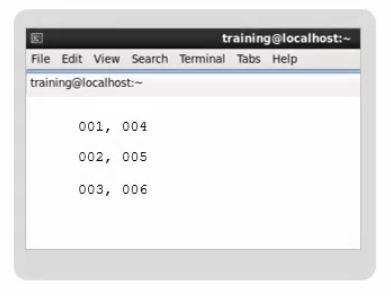Some of the functions that can be performed with Double RDDs are:

• Distinct

• Sum

RDDs can be created from a text file or data in memory.

Let us first learn how to create RDD from a Text File.

## How to create RDD from a Text File?

• To create a file-based RDD, you can use the command SparkContext.textFile or sc.textfile and pass one or more file names. For example, to create RDD based on the file simplilearn.txt, you can use sc.textfile and provide the filename simplilearn.txt in brackets and within quotes as demonstrated in the below example.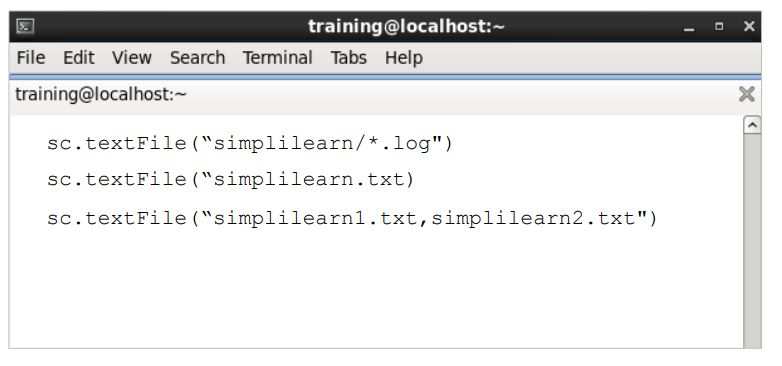• To create RDD based on a comma-separated list of files, simplilearn1.txt and simplilearn2.txt, or wildcard list of files, you must provide the filenames in the sc.textfile command as shown in the example.

Given below is an example that shows a text file simplilearn.txt used to create an RDD.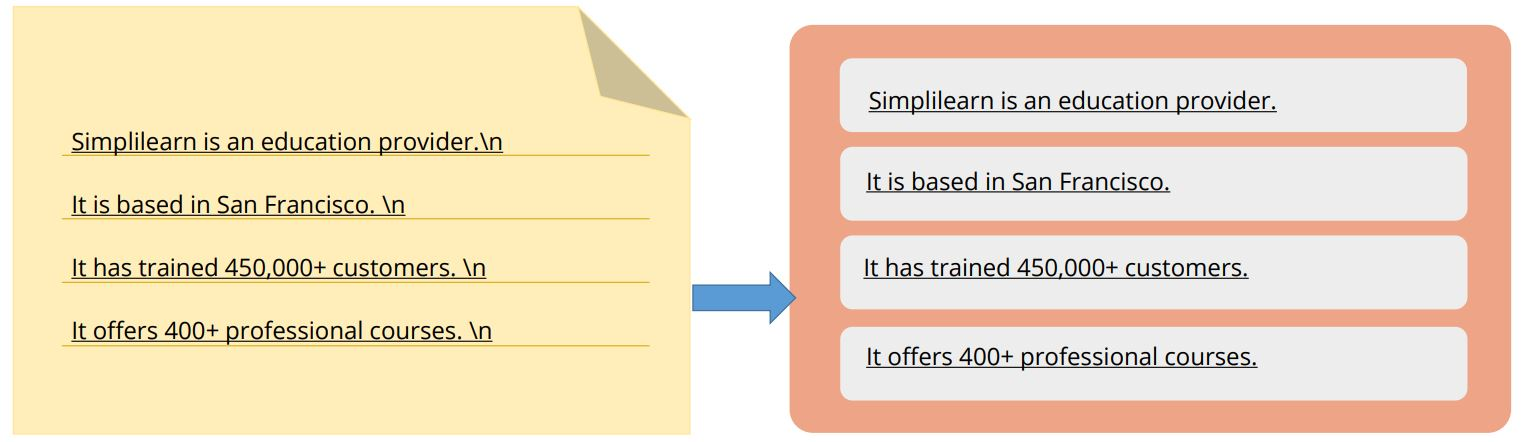Each line in the text is considered a separate RDD element. Another point to note is that text File works only with line delimited text files.

Note that each line in the text file is a separate record in the RDD. The text File command maps each line in the file to a separate RDD element.

Let us now learn how to create RDD from Collections.

## Creating RDD from Collections

To create RDD from a collection, you use the sc.parallelize and pass the collection.

Given below is an example that shows a collection of four words being created by name data.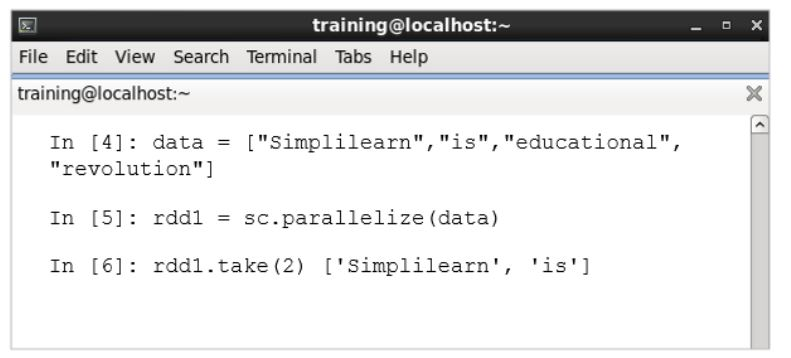The RDD named rdd1 is then created by passing this collection to sc.parallelize. Then, two elements of the collection, which are the words Simplilearn and is, are printed by using the take action.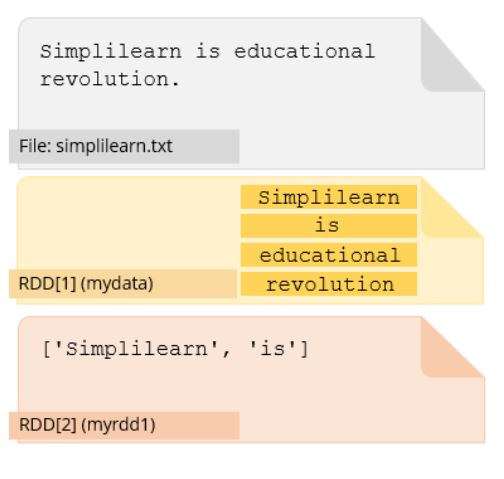Let us now learn how to create RDD from Whole Files.

## Creating RDD from Whole Files

We have previously seen that sc.textfile maps each line in a file to a separate RDD element. However, the files such as JSON or XML have a multi-line input format.

To deal with such files, sc.wholeTextFiles must be used, and the directory name must be passed. The entire contents of each file in the specified directory are then mapped to a single RDD element.

However, do note that this works only for small files as the memory capacity must be sufficient for the element.

In the example given below, file1.json and file2.json separate files in a single directory. All the files are then combined into one RDD.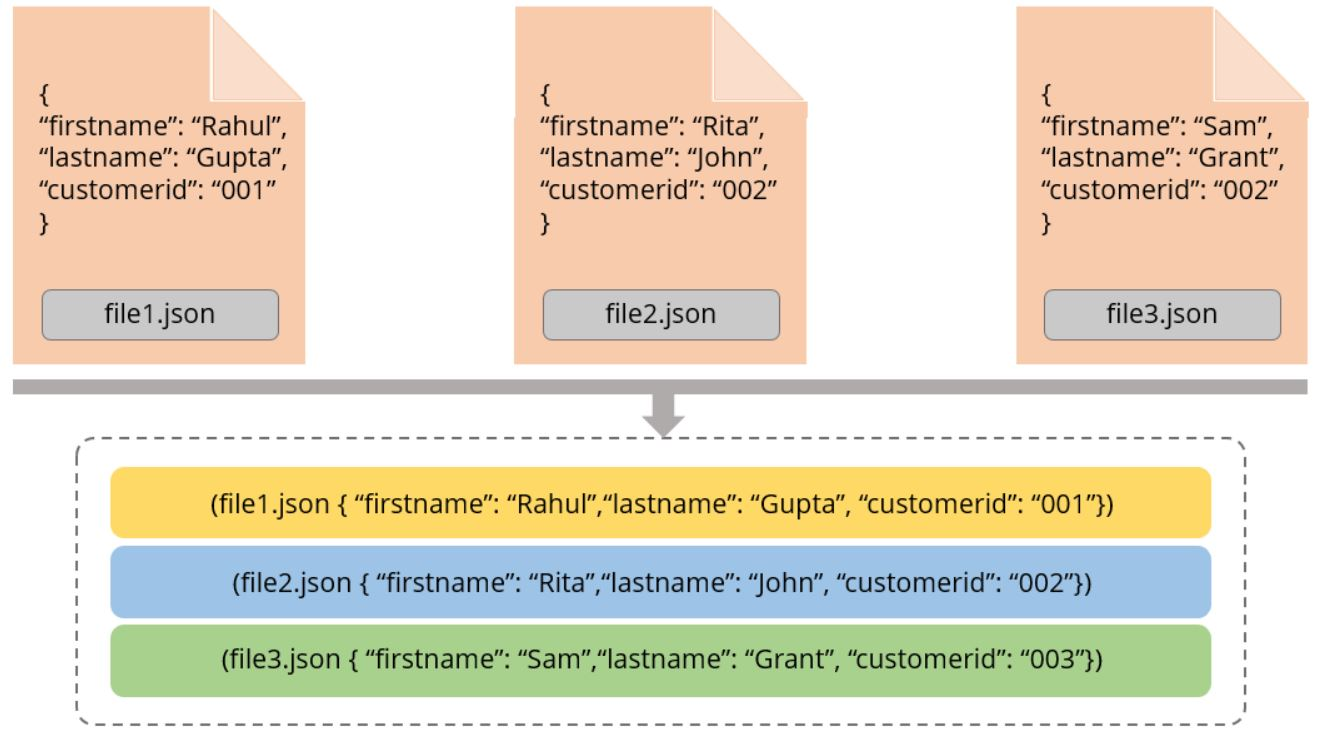In the next section, we will discuss creating pair rdds.

## Creating Pair RDDs

The first step in most workflows is to get the data into key/value form and so it is essential to determine what the RDD should be keyed on and what value the key will hold. So, a Pair RDD is created.

To create a Pair RDD, use functions such as Map, flatMap/flatMapValues, and keyBy.

In the example given below, an RDD named “users” is created by reading the text file.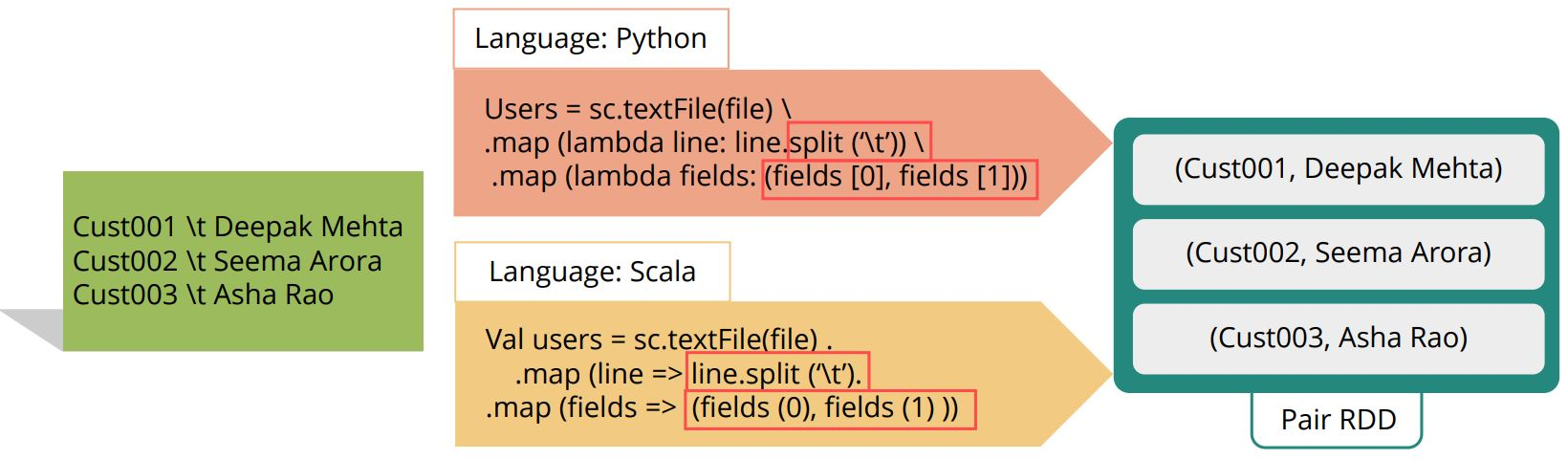The text in the file “Simplilearn” is separated with the delimiter \t using the split function. It is then transformed into the key-value format, and a Pair RDD is created.

Next, we will learn about input and output formats supported by Spark.

## Input and Output Formats

Spark RDDs can also reference Hadoop InputFormat and OutputFormat Java, classes.

An example of core Hadoop class for newline-delimited text files includes:

• TextInputFormat

• TextOutputFormat

Other examples include:

• SequenceInputFormat

• SequenceOutputFormat

• FixedLengthInputFormat

Spark also supports many implementations available in additional libraries such as:

• AvroKeyInputFormat

• AvroKeyOutputFormat in the Avro libraryIn the next section, let us look at the operations that can be performed on RDDs.

## Operations in RDDs

Single-RDD and multi-RDD transformations are two types of operations that can be executed on RDDs.

Some of the single-RDD transformations include:

• Map: Map is a transformation that applies a transformation function on each item of the RDD and returns the result as a new RDD.

• FlatMap: FlatMap is a transformation that is similar to map, but can map one element in the base RDD to multiple elements.

• Distinct: is a transformation that returns a new RDD that contains each unique value only once.

• SortBy: SortBy is a transformation that is used for sorting.

Some of the multi-RDD transformations include:

• Union: Union is a multi-RDD transformation that performs the standard set operation of A Union B.

• Intersection: Intersection creates a new RDD with all elements in both original RDDs.

• Zip: Zip is a transformation that joins two RDDs by combining each line of either partition with the corresponding line in the other.

Let us look at an example showing the use of flatMap and distinct in Python and Scala languages.

### Example of Single-RDD Transformation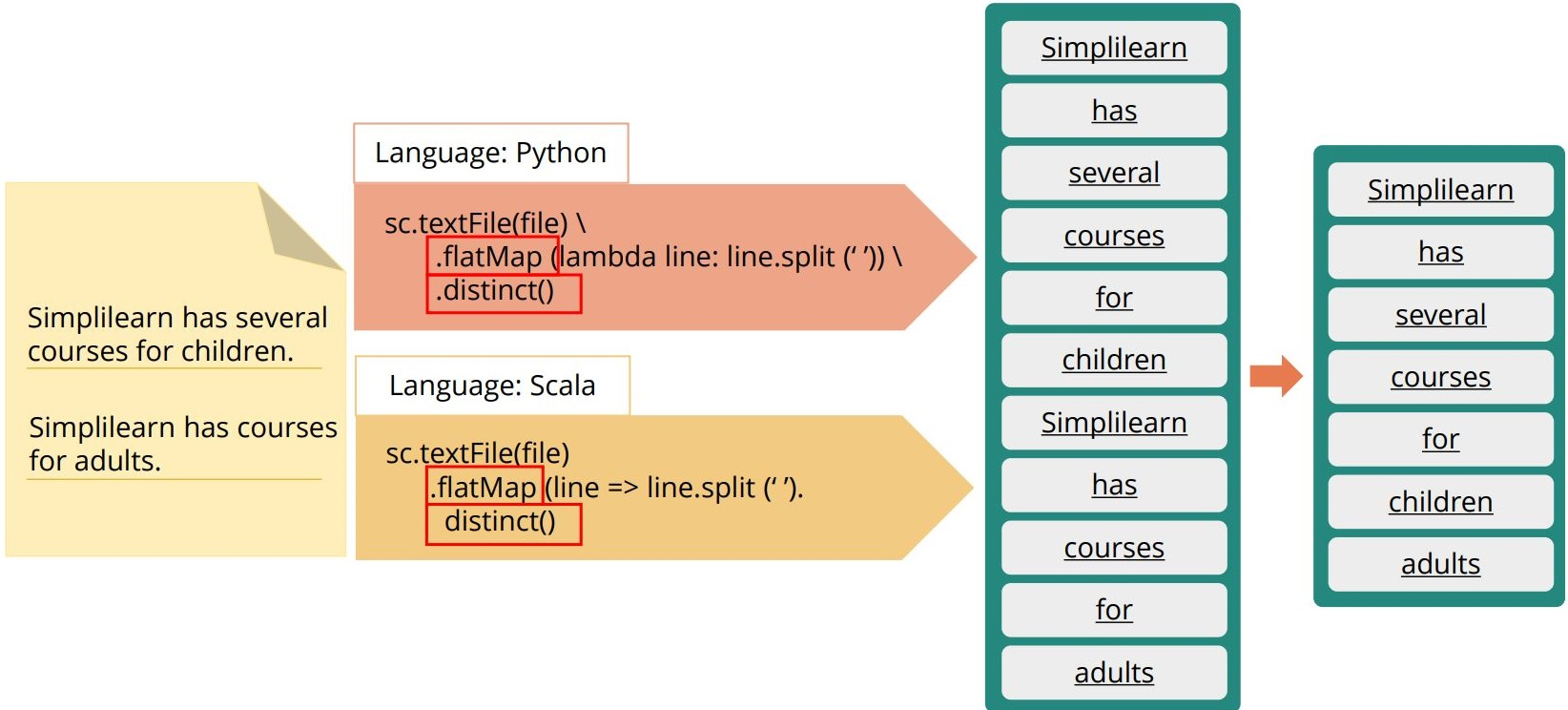Each item of the RDD is returned as a separate RDD. The application of distinct makes sure each item is returned only once.

Here is an example using subtract, zip, and union.

### Example of Double-RDD Transformation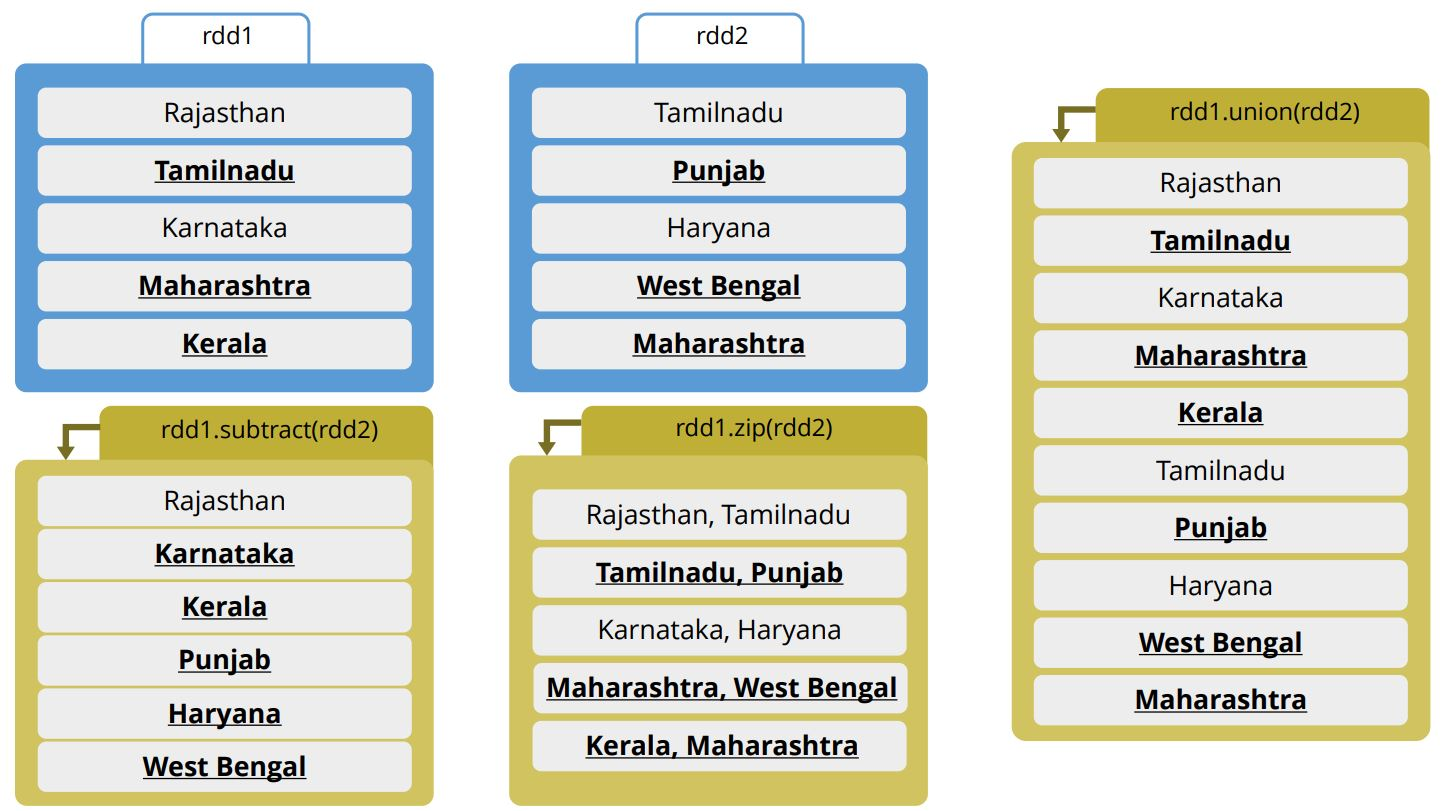• Subtract eliminates the common terms between rdd1 and rdd2 and outputs the remaining terms in rdd1.

• Zip combines each line of rdd1 with the corresponding line in rdd2.

• Union combines rdd1 and rdd2 and outputs the entire list of terms from both the RDDs in a single RDD.

### More RDD Operations

There are more RDD operations that allow sampling, retrieving statistical data, and so on. Let us discuss them briefly.

Sampling operations include:

• Sample: Sample randomly selects a fraction of the items of an RDD and returns them in a new RDD

• TakeSample: TakeSample which returns an array of sampled elements

• SampleByKey: SampleByKey which randomly samples the key-value pair RDD according to the fraction of each key you want to appear in the final RDD.

Some of the Double RDD operations include Statistical functions such as:

• Mean

• Sum

• Variance

• Stdev

These can help in the computation of statistical values.

Other RDD operations include:

• Collect: It prints all RDD elements on the console. First, which returns the first element of RDD.

• Foreach: It applies a function to each element of RDD.

Let us now look at operations that can be performed on Pair RDDs.

### Pair RDD Operations

Some of the important ones are:

• CountByKey: It returns a map with the count of occurrences of each key.

• GroupByKey: It groups all the values for each key in an RDD.

• SortByKey: It sorts data in ascending or descending order.

• Join: It returns an RDD containing all pairs with matching keys from two RDDs.

Let us understand sortByKey and groupByKey operations with an example.

### SortByKey and GroupByKey Operations

In the example shown below, we have a Pair RDD named prdd1.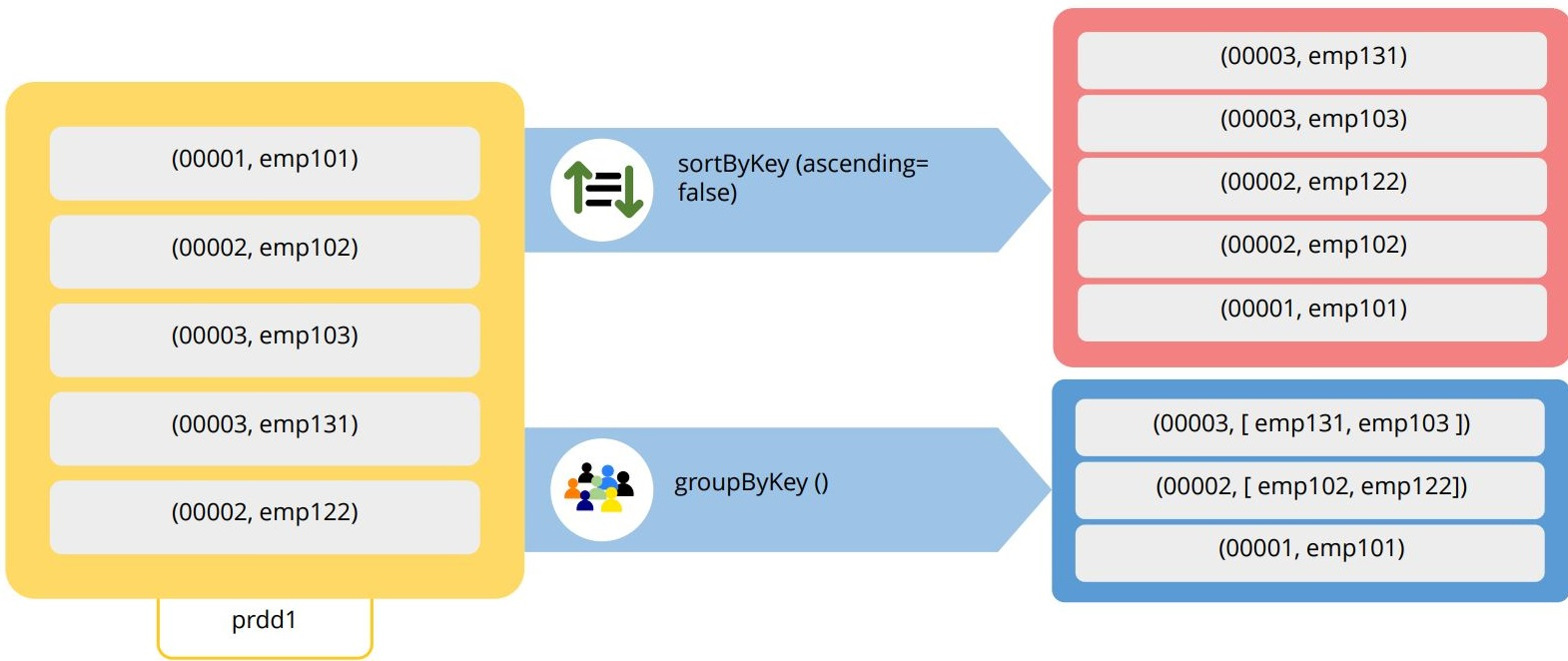When you execute the sortByKey operation on it by specifying ascending as “false,” observe that it sorts the RDD in descending order by considering the first value as the key.

When you execute the groupByKey operation on it, values for each key are grouped. Thus, for the key 00002, the values emp912 and emp331 are grouped.

Let us now look at the join operation.

### Join operation

In the example on shown below, we have two Pair RDDs, sale profit and sales year.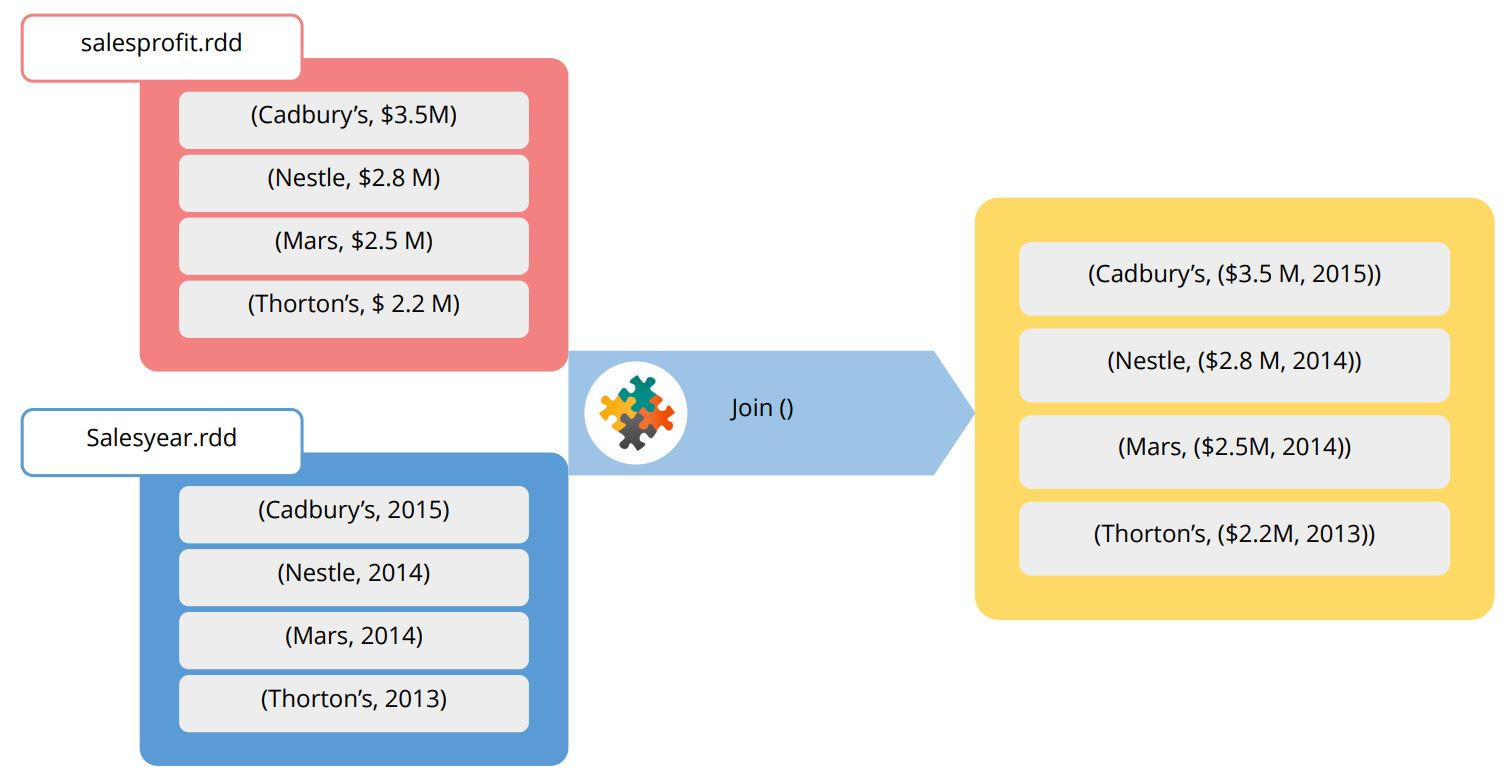When the join operation is executed, the product name is considered the key. The matching keys from the two RDDs are searched for, and the pairs are returned as a single RDD.

## Summary

Let’s now summarize what we learned in this lesson.

• RDDs can be created from file, collections, and from other RDDs.

• All types of data are supported by generic RDDs.

• Transformations supported by Spark include single-RDD and multi-RDD transformations.

• Some of the transformations include a map, flatMap, union, intersection, distinct, and so on.

• Sampling and Statistical operations are also supported by Spark.

Preparing to become an expert in Big Data Hadoop and Spark Developer ? Check out the course here!

## Conclusion

This concludes the lesson on RDDs in Spark. In the next lesson, we will look at ‘Implementation of Spark Applications

### Find our Big Data Hadoop and Spark Developer Online Classroom training classes in top cities:

Name Date Place
Big Data Hadoop and Spark Developer 6 Feb -20 Mar 2021, Weekend batch Your City View Details
Big Data Hadoop and Spark Developer 6 Mar -17 Apr 2021, Weekend batch Dallas View Details
Big Data Hadoop and Spark Developer 19 Mar -30 Apr 2021, Weekdays batch New York City View Details
Related Courses
Learner Reviews
Related Articles
• Disclaimer
• PMP, PMI, PMBOK, CAPM, PgMP, PfMP, ACP, PBA, RMP, SP, and OPM3 are registered marks of the Project Management Institute, Inc.

Name*
Email*
Phone Number*